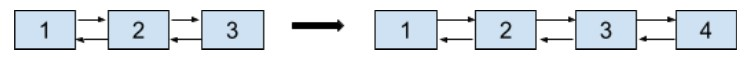Problem title
Difficulty
Avg time to solve

Hourglass Pattern
Moderate
--
Word Search - ll
Moderate
30 mins
Distribute Items
Moderate
31 mins
Amazing Strings
Easy
--
Reverse string Word Wise
Moderate
--
Binary to decimal
Easy
--
Triangle Star Pattern
Easy
--
Rotate Matrix K times
Moderate
--
Print Diagonal
Easy
--
Easy
15 mins

# Insertion In Doubly Linked List

Difficulty: EASY
Avg. time to solve
15 min
Success Rate
95%

Problem Statement

#### Note:

``````The position given will always be less than or equal to the length of the linked list.
Assume that the Indexing for the linked list starts from 0.
``````
##### EXAMPLE:
``````Input :
‘K’ = 3, ‘VAL’ = 4
list = [1, 2, 3]
Output: [1, 2, 3, 4]
````````````The ‘VAL’ = 4, is inserted at end of the above doubly linked list.
``````
##### Input Format :
``````The first line will contain the integer 'T', the number of test cases.

The first line of each test case contains the integers ‘K’, and ‘VAL’, denoting the position of insertion operation, and the value of the new node to be inserted in the list respectively.

The second line of each test case contains an array of positive integers denoting the nodes of the linked list.
``````
##### Output format :
``````For each test case, print the doubly linked list after the insertion operation.
``````
##### Note :
``````You don't need to print anything. It has already been taken care of. Just implement the given function.
Here, '-1' marks the end of the linked list.
``````
##### Constraints :
``````1 <= 'T' <= 10
1 <= Length of the list <= 10^4
0 <= ‘K’ <= Length of the list, where ‘K’ is 0-indexed.
1 <= 'VAL' <= 10^5

Time Limit: 1 sec
``````
##### Sample Input 1:
``````2
0 0
1 2 3 4 -1
5 5
5 4 3 2 1 -1
``````
##### Sample Outpu.t 1:
``````0 1 2 3 4
5 4 3 2 1 5
``````
##### Explanation Of Sample Input 1 :
``````For the first test case, ‘K’ = 0 denotes the starting index of the list, so the new element is inserted before the head of the list and the new head will be then a node with ‘VAL’ = 0

Hence, the resulting doubly linked list would be : 0 1 2 3 4

For the second test case, ‘K’ = 5 = size of the list denotes the new element to be inserted at the end of the list.

Hence, the resulting doubly linked list would be: 5 4 3 2 1 5
``````
##### Sample Input 2 :
``````2
2 4
10 11 5 -1
1 5
2 8 -1
``````
##### Sample Output 2 :
``````10 11 4 5
2 5 8
``````Console